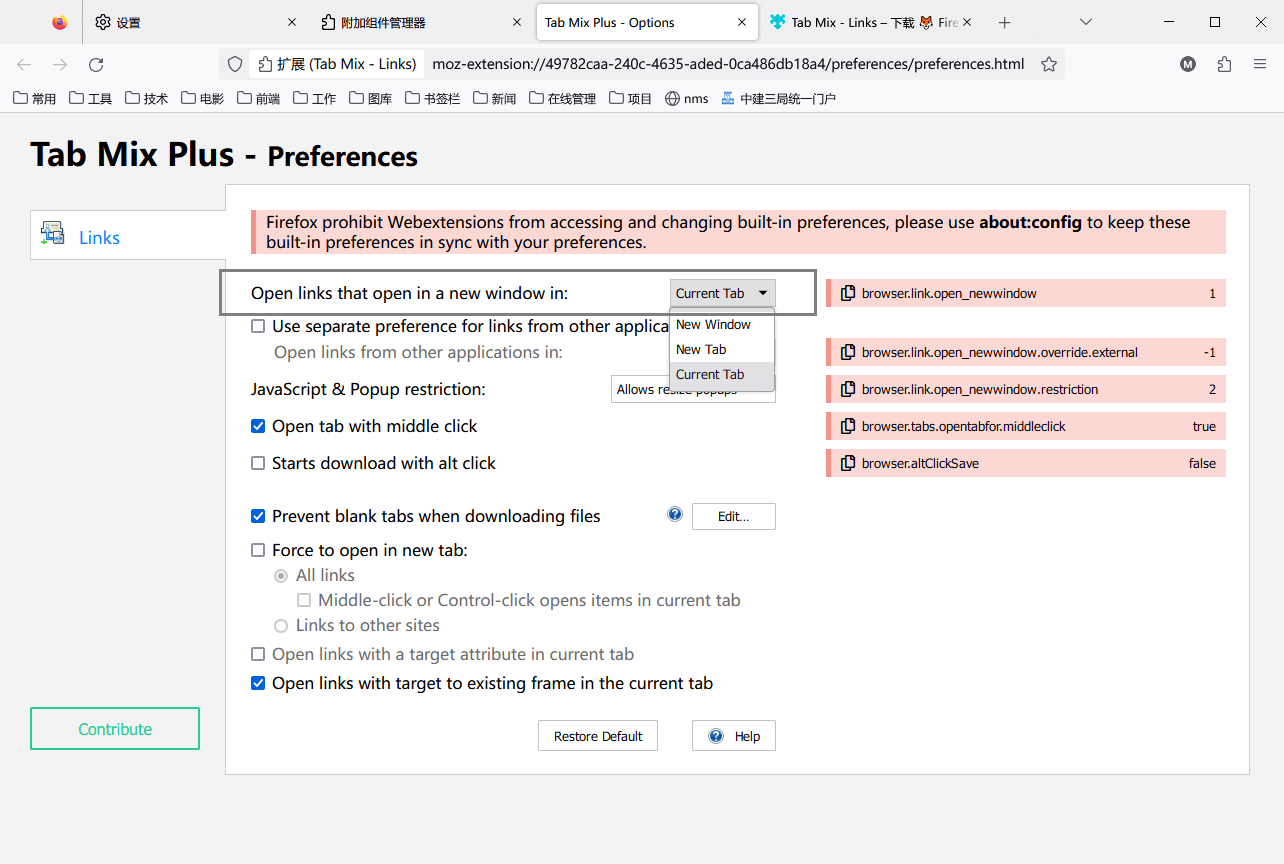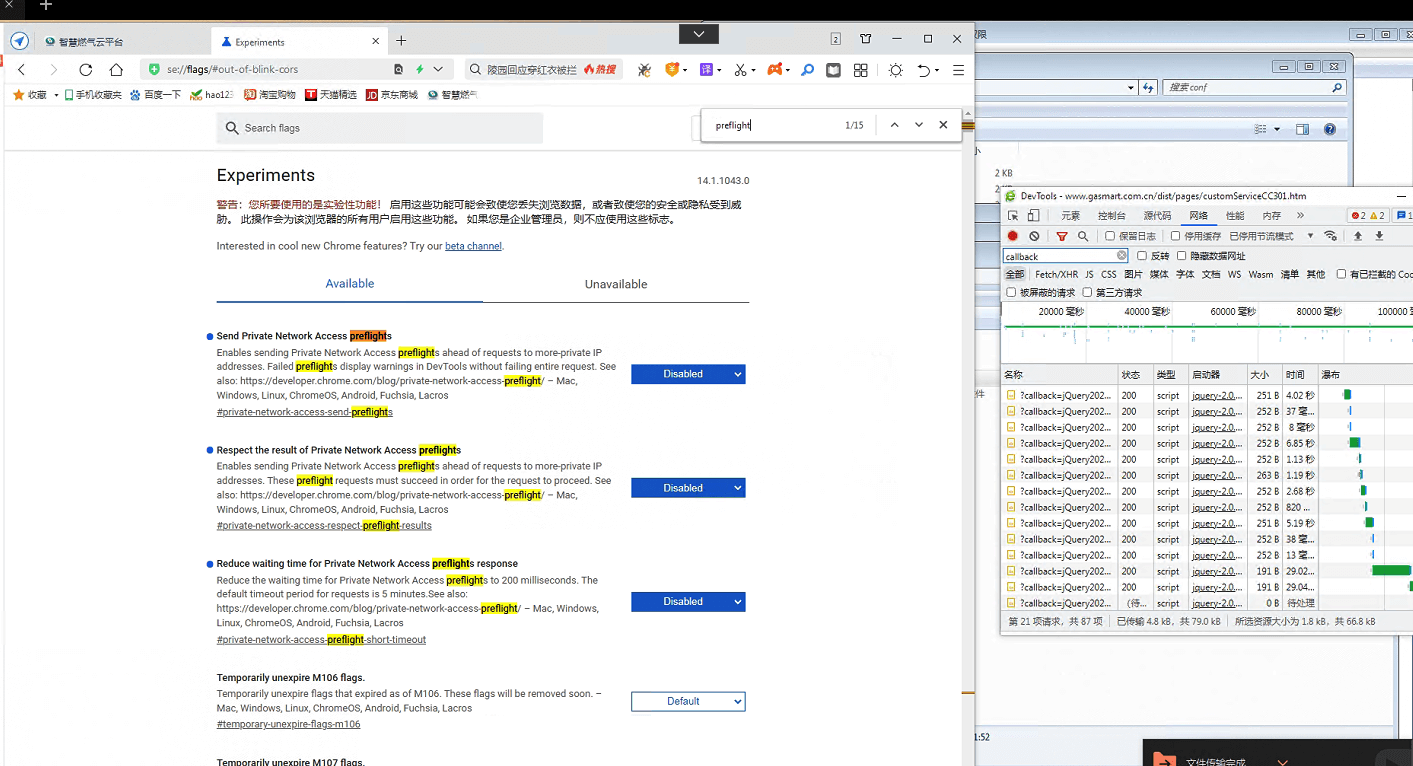## indexOf 与 for 循环性能分析

``````console.clear()
let a = []
// populating array data
for (let i = 0; i < 100000; i++) {
a.push(i)
}
let testNum = 90000
let found
let totalMS4ForLoop = 0
let totalMS4ForIn = 0
let totalMS4IndexOf = 0
let start
let end
// simulating 10000 requests which are come consecutively
for (o = 0; o < 10000; o++) {
start = Date.now()
for (let i = 0; i < a.length; i++) {
if (a[i] == testNum) { found = a[i]; break }
}
end = Date.now()
totalMS4ForLoop += end - start
start = Date.now()
for (let j in a) {
if (a[j] == testNum) { found = a[j]; break }
}
end = Date.now()
totalMS4ForIn += end - start
start = Date.now()
found = a[a.indexOf(testNum)]
end = Date.now()
totalMS4IndexOf += end - start
}
console.log("100000x10000 for-loop executions took total " + totalMS4ForLoop + " ms.")
console.log("100000x10000 for-in executions took total " + totalMS4ForIn + " ms.")
console.log("100000x10000 indexOf executions took total " + totalMS4IndexOf + " ms.")``````

100000x10000 for-loop executions took total 283 ms.
100000x10000 for-in executions took total 33368 ms.
100000x10000 indexOf executions took total 113 ms.

## 使用JS，判断是一个变量是否为数字或字符串数字

``````function isNumeric(value) {
// 使用 typeof 来判断变量是否为数字类型
if (typeof value === 'number') {
return true;
}

// 使用正则表达式来检查字符串是否表示数字
if (typeof value === 'string' && value.trim() !== '') {
// 去除字符串两端的空格，并检查是否为空字符串
return /^\d+(\.\d+)?\$/.test(value.trim());
}

return false;
}``````

IDEA端口占用，而实际没有占用

## Chrome浏览器的 Ctrl+W 关闭网页的快捷键失效

Chrome浏览器的 Ctrl+W 关闭网页的快捷键经常用着用着不好使了，原因是企业微信的导致的快捷键冲突，关闭企业微信或其他企业微信私有部署的软件，就正常了。记录一下。

## 开启浏览器的单标签模式## js 数组 倒序

``const arr = [1, 2, 3, 4, 5];``

``arr.reverse();``

``````const arr = [1, 2, 3, 4, 5];
const reversedArr = arr.slice().reverse();``````

## windows chrome 浏览器 关闭 preflight 预检策略

Chrome 浏览器的 CORS 预检请求（Cross-Origin Resource Sharing Preflight Request），在 Chrome 浏览器中关闭这些预检请求，使用以下方法：## JAVA中对List根据Map某个key值进行排序

### JAVA中对List根据Map某个key值进行排序

1. 使用Stream API将List转换为Stream。
2. 使用sorted方法对Stream中的元素进行排序，传入Comparator.comparing方法，并使用Lambda表达式指定要排序的Map中的key值。
3. 使用collect方法将Stream转换为List。

``````List<Map<String, Object>> list = new ArrayList<>();
// 假设list中有多个Map元素，每个Map都包含“name”和“age”两个key值

List<Map<String, Object>> sortedList = list.stream()
.sorted(Comparator.comparing(m -> (String) m.get("name")))
.collect(Collectors.toList());
// 根据Map中的“name”值对List进行排序``````

### JAVA中对List根据Map某个key值按照指定list顺序进行排序

1. 创建一个包含指定顺序的key值列表。
2. 使用Stream API将List转换为Stream。
3. 使用sorted方法对Stream中的元素进行排序，传入一个Comparator对象，并使用Lambda表达式指定排序的规则。在Lambda表达式中，首先使用indexOf方法获取当前Map的key值在指定顺序列表中的下标，然后使用compareTo方法对下标进行比较。
4. 使用collect方法将Stream转换为List。

``````List<Map<String, Object>> list = new ArrayList<>();
// 假设list中有多个Map元素，每个Map都包含“name”和“age”两个key值

List<String> order = Arrays.asList("Tom", "Jerry", "Mike", "John");
// 假设按照指定顺序排序的key值为“name”，顺序为“Tom”、“Jerry”、“Mike”、“John”

List<Map<String, Object>> sortedList = list.stream()
.sorted(Comparator.comparing(m -> order.indexOf(m.get("name"))))
.collect(Collectors.toList());
// 根据Map中的“name”值按照指定顺序对List进行排序``````